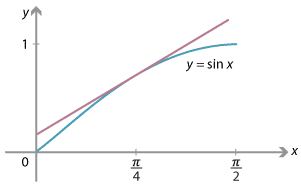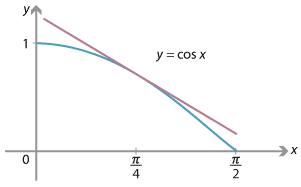## Content

### Differentiating trigonometric functions

#### The derivative of sine

Since the graph of $$y = \sin x$$ is a smooth curve, we would like to find the gradient of the tangent to the curve at any point on it.

Before doing this, we derive a useful trigonometric identity that will assist us.

Using the compound-angle formulas, we have

\begin{align*} \sin(A+B) - \sin(A-B) &= \sin A\,\cos B + \cos A\,\sin B - (\sin A\,\cos B - \cos A\,\sin B) \\ &= 2\,\cos A\,\sin B. \end{align*} If we put $$C=A+B$$ and $$D=A-B$$, we can add these equations to obtain $$A=\dfrac{1}{2}(C+D)$$ and subtract them to obtain $$B=\dfrac{1}{2}(C-D)$$. Substituting these back, we obtain the sine difference formula: $\sin C - \sin D = 2\,\cos\Bigl(\dfrac{C+D}{2}\Bigr)\,\sin\Bigl(\dfrac{C-D}{2}\Bigr).$

To find the derivative of $$\sin x$$, we return to the first principles definition of the derivative of $$y=f(x)$$:

$\dfrac{dy}{dx} = \lim_{h\to 0} \dfrac{f(x+h)-f(x)}{h}.$

Substituting $$y=\sin x$$, we have

$\dfrac{dy}{dx} = \lim_{h\to 0} \dfrac{\sin(x+h) - \sin x}{h}.$

Applying the sine difference formula, we have

$\lim_{h\to 0} \dfrac{\sin(x+h) - \sin x}{h} = \lim_{h\to 0} \dfrac{2\,\cos\bigl(x+\dfrac{h}{2}\bigr)\,\sin\bigl(\dfrac{h}{2}\bigr)}{h}.$

We can put $$u=\dfrac{h}{2}$$. Then $$u\to 0$$ as $$h\to 0$$. So the limit becomes

\begin{align*} \dfrac{dy}{dx} &= \lim_{u\to 0} \dfrac{2\,\cos(x+u)\,\sin u}{2u} \\ &= \Bigl(\lim_{u\to 0} \cos(x+u)\Bigr) \times \Bigl(\lim_{u\to 0}\dfrac{\sin u}{u}\Bigr) \\ &= \cos x \times 1 \\ &= \cos x. \end{align*}

We can thus conclude that

$\dfrac{d}{dx} (\sin x) = \cos x.$

This result is both simple and surprising, and students need to commit it to memory.

The derivation above involved a number of ingredients and is often difficult for students the first time through.

#### Derivatives of other trigonometric functions

Now that the derivative of sine is established, we can use the standard rules of calculus — the chain, product and quotient rules — to proceed.

Since $$\cos x = \sin\bigl(x + \dfrac{\pi}{2}\bigr)$$, we can apply the chain rule to see that

\begin{align*} \dfrac{d}{dx} (\cos x) &= \dfrac{d}{dx} \Bigl( \sin\bigl(x + \dfrac{\pi}{2}\bigr)\Bigr) \\ &= \cos \bigl(x + \dfrac{\pi}{2}\bigr) \\ &= -\sin x. \end{align*}

Thus

$\dfrac{d}{dx}(\cos x) = -\sin x.$

This is also a simple and surprising result that needs to be committed to memory.

The following graphs illustrate what is happening geometrically. If we draw the tangent to the curve $$y=\sin x$$ at a point with $$0 < x < \dfrac{\pi}{2}$$, then the tangent clearly has positive gradient, while a tangent to $$y=\cos x$$, in the same range, clearly has negative gradient.Detailed descriptionDetailed description

The derivative of $$\cos x$$ can also be found by using first principles.

Exercise 4

1. Show that $\cos C - \cos D = -2\,\sin\Bigl(\dfrac{C+D}{2}\Bigr)\,\sin\Bigl(\dfrac{C-D}{2}\Bigr).$
2. Show by first principles that $\dfrac{d}{dx}(\cos x) = -\sin x.$

Exercise 5

By writing $$\tan x = \dfrac{\sin x}{\cos x}$$ and applying the quotient rule, prove that

$\dfrac{d}{dx} (\tan x) = \sec^2 x.$

Students need to remember the derivatives of $$\sin$$, $$\cos$$ and $$\tan$$.

The rules of calculus can also be used to find the derivatives of the reciprocal functions.

Exercise 6

Show that

1. $$\dfrac{d}{dx} (\operatorname{cosec} x) = -\operatorname{cosec} x\,\cot x$$
2. $$\dfrac{d}{dx} (\sec x) = \sec x\,\tan x$$
3. $$\dfrac{d}{dx} (\cot x) = -\operatorname{cosec}^2 x$$.

These three derivatives need not be committed to memory.

#### Example

Use the rules of calculus to differentiate each of the following functions with respect to $$x$$:

1. $$4\,\sin(2x^2)$$
2. $$x\,\cos(2x)$$
3. $$e^{3x}\tan(4x)$$.

#### Solution

1. $$\dfrac{d}{dx}\bigl(4\,\sin(2x^2)\bigr) = 16x\,\cos(2x^2)$$
2. $$\dfrac{d}{dx}\bigl(x\,\cos(2x)\bigr) = -2x\,\sin(2x)+\cos(2x)$$
3. $$\dfrac{d}{dx}\bigl(e^{3x}\tan(4x)\bigr) = 4e^{3x}\sec^2(4x) + 3e^{3x}\tan(4x)$$.

Exercise 7

Show that

$\dfrac{d}{dx} \log_e\Bigl(\dfrac{1+\sin x}{\cos x}\Bigr) = \sec x.$

Next page - Content - Applications of the derivatives